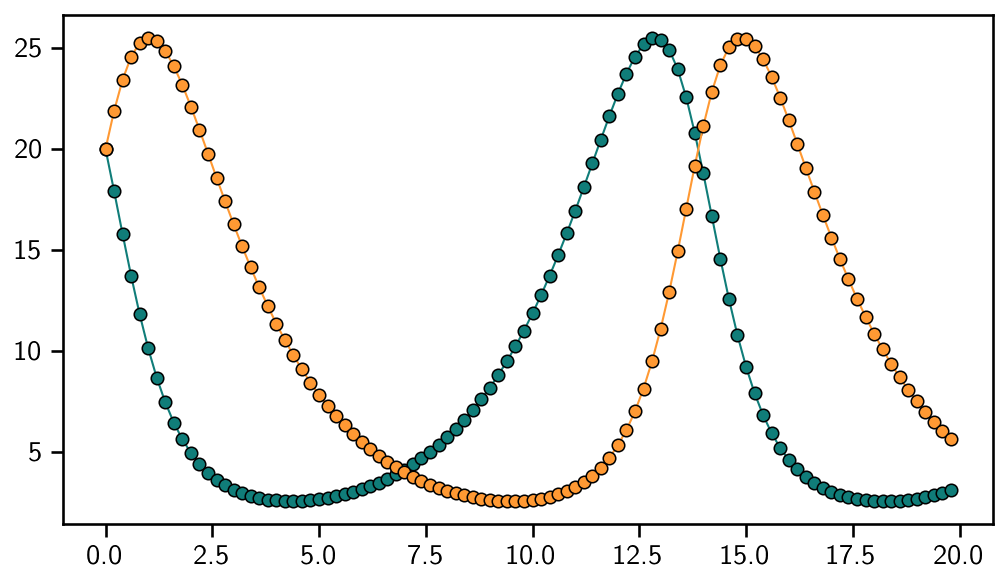# Ordinary Differential Equations¶

The behaviour of complex systems which evolve over time is often described via the use of ordinary differential equations. ProbNum provides a set of methods to solve ordinary differential equations based on filtering approaches which quantify the uncertainty introduced by discretization.

See the notebooks below to learn more about how to use ProbNum’s functionality for ordinary differential equations.You can also interactively try out thedirectly in the browser or by downloading the notebooks from the GitHub repository.